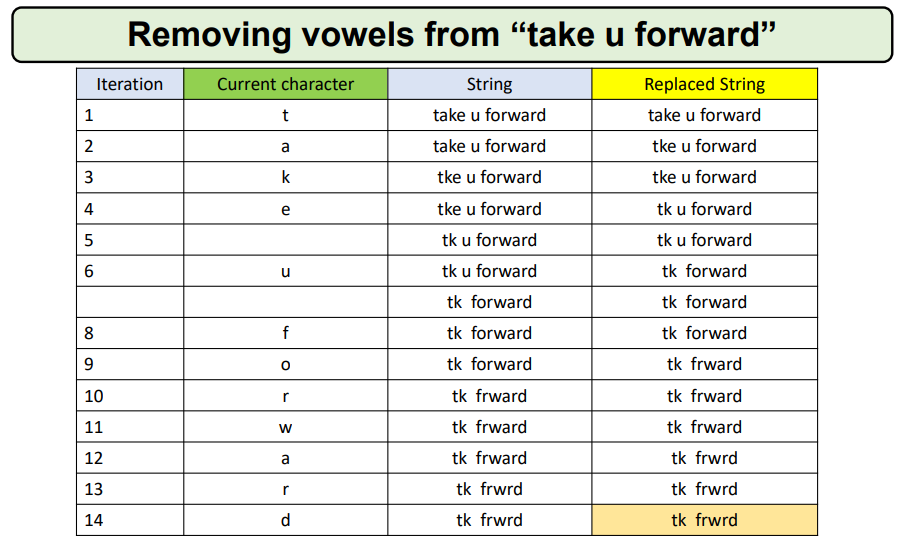# Remove all vowels from the String

Problem Statement: Given a String, write a program to remove vowels from a given String.

Examples:

```Example 1:
Input: Str = “take u forward”
Output: tk  frwrd
Explanation: All vowels are removed from the given String.

Example 2:
Input: Str = “I am very happy today”
Output:  m vry happy tdy
Explanation: All vowels are removed from the given String.```

### Solution

Disclaimer: Don’t jump directly to the solution, try it out yourself first.

Approach:  Go through every character of the string, and if a vowel is found then replace it with a removed vowel string.

For Eg,

Str = “take u forward”Code:

## C++ Code

``````#include <iostream>
#include <string.h>
using namespace std;
// Function to remove vowels from a string
string RemoveVowels(string str)
{
for (int i = 0; i < str.length(); i++)
{
if (str[i] == 'a' || str[i] == 'e' || str[i] == 'i' || str[i] == 'o' || str[i] == 'u' || str[i] == 'A' || str[i] == 'E' || str[i] == 'I' || str[i] == 'O' || str[i] == 'U')
{
str = str.substr(0, i) + str.substr(i + 1);
i--;
}
}
return str;
}
int main()
{
string str = "take u forward";
cout <<"String after removing the vowels \n" <<RemoveVowels(str) << endl;
return 0;
}
``````

Output:

String after removing the vowels
tk frwrd

Time Complexity: O(n) since the total number of iterations required is the number of characters in a string

Space Complexity: O(1)

## Java Code

``````import java.util.*;
public class Main {
// Function to remove vowels from a string
public static String RemoveVowels(String str) {
for (int i = 0; i < str.length(); i++) {
if (str.charAt(i) == 'a' || str.charAt(i) == 'e' || str.charAt(i) == 'i' || str.charAt(i) == 'o' || str.charAt(i) == 'u' || str.charAt(i) == 'A' || str.charAt(i) == 'E' || str.charAt(i) == 'I' || str.charAt(i) == 'O' || str.charAt(i) == 'U') {
str = str.substring(0, i) + str.substring(i + 1);
i--;
}
}
return str;
}

public static void main(String[] args) {
String str = "take u forward";
System.out.println("String after removing the vowels \n"+RemoveVowels(str));
}
}
``````

Output:

String after removing the vowels
tk frwrd

Time Complexity: O(n) since the total number of iterations required is the number of characters in a string

Space Complexity: O(1)

Special thanks to Gurmeet Singh for contributing to this article on takeUforward. If you also wish to share your knowledge with the takeUforward fam, please check out this article